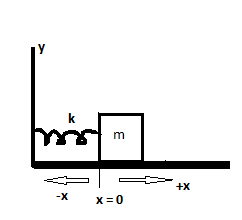# A spring and block are in the arrangement as shown in the figure. When the block is pulled out to...

## Question:

A spring and block are in the arrangement as shown in the figure. When the block is pulled out to x = +5.0 cm, we must apply a force of magnitude 360 N to hold it there. We pull the block to x = 12.0 cm and then release it. How much work does the spring do on the block when the block moves from xi = +6.0 cm to (a) x = +3.0 cm, (b) x = -3.0 cm, (c) x= -6.0 cm, and (d) x = -10.0 cm?## Work done by spring:

If a block moves a distance due to spring force (F=-kx) then work done by spring force is

{eq}\Rightarrow W=\int_{x_i}^{x_f}-kx\Delta x\\ \Rightarrow W=\frac{1}{2}K(x_i^2-x_f^2) {/eq}

Become a Study.com member to unlock this answer! Create your account

At x=+5 cm we hold the block by F=360 N

so we can write

{eq}F=Kx \Rightarrow 360=K\times .05\\ \Rightarrow K=72 \ N/m {/eq}

We know workdone by...Hooke's Law & the Spring Constant: Definition & Equation

from

Chapter 4 / Lesson 19
203K

After watching this video, you will be able to explain what Hooke's Law is and use the equation for Hooke's Law to solve problems. A short quiz will follow.